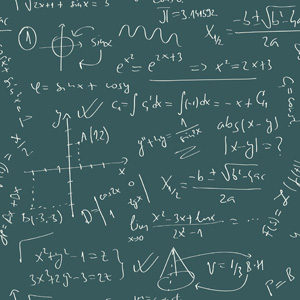#Mathematics Transfer Degree Program

The Math program provides the fundamental mathematics courses to prepare general students and math and engineering majors for transfer to a four-year institution.

A student’s class choices should be planned with the requirements of the transfer institution in mind.  See your advisor for enrollment information and transfer institution requirements.

Most students who decide to major in Mathematics at a four-year institution will plan a course of study that will lead to an Associate in Science Degree.

Recommended Courses for Students Planning to Major in Mathematics

Course Code Course Title Credit Hrs.
MATH-115 Calculus with Analytic Geometry I 5
MATH-120 Calculus with Analytic Geometry II 5
MATH-201 Calculus with Analytic Geometry III 3
MATH-202 Differential Equations 3
ENGR-210 Engineering Physics I 5
ENGR-211 Engineering Physics II 5
A Computer Programming Course 3

To complete an associate degree the program emphasis credit hours and the general education credit hours, which must be taken from the list approved by the General Education Committee, must total at least 64 credit hours.

Description of Courses

MATH-102. Intermediate Algebra*. 3 Hours. This course is designed to survey the basic topics in algebra which will enable the student to enter a course in college algebra. Prerequisite: A minimum score of 250 on the ACCUPLACER test or credit in Introductory Algebra with a grade of C or above.

MATH-104. College Algebra with Review*. 5 Hours. Topics included in the course are quadratic equations, ratio, proportion and variation, complex numbers, determinants, and elimination theory and inequalities. Prerequisite: A minimum score of 263 on the ACCUPLACER test or credit in Intermediate Algebra with a grade of C or above.

MATH-105. College Algebra*. 3 Hours. Topics included in the course are quadratic equations, ratios, proportions and variation, complex numbers, determinants, and elimination theory and inequalities. Prerequisite: A minimum score of 263 on the ACCUPLACER test or credit in Intermediate Algebra with a grade of C or above.

MATH-106. Trigonometry*. 3 Hours. This course is an introduction to trigonometry for students of various majors. Topics included in this course are law of sines, law of cosines, and the Pythagorean Theorem. Prerequisite: A minimum score of 263 on the ACCUPLACER test or credit in College Algebra with a grade of C or above.

MATH-115. Calculus with Analytic Geometry I*. 5 Hours. This course is a study of derivatives and integrals with applications. Prerequisite: Trigonometry with a grade of C or above, or ACCUPLACER score of 263 or above on College Algebra test.

MATH-117.  Introduction to Analytic Processes. 3 Hours.  This course employs the concepts of function, limit, derivative, integral and related topics.  This three-hour course is designed for the non-mathematics or non-engineering majors; pre-calculus for business majors.  Prerequisite:  College Algebra with a grade of C or above.

MATH-120.  Calculus with Analytic Geometry II. 5 Hours.  This course treats integration techniques, series, polar coordinates and vector applications.  Prerequisite:  Calculus with Analytic Geometry I with a grade of C or above.

MATH-201.  Calculus with Analytic Geometry III. 3 Hours.  This course treats the multi-variable concepts of partial derivatives and multiple integrals, vector valued functions and line integrals.  Prerequisite:  Calculus with Analytic Geometry II with a grade of C or above.

MATH-202.  Differential Equations.  3 Hours.  This course treats the various types of solutions of differential equations.  Prerequisite:  Calculus with Analytic Geometry III with a grade of C or above.

MATH-250.  Elementary Statistics.  3 Hours.  This course is an introduction to statistics for students of various majors.  Topics included in this course are analysis of data, discrete and continuous distributions, sampling and statistical inference.  Prerequisite: Intermediate Algebra with a grade of C or above.

*Completion of the ACCUPLACER Test is required for students prior to enrolling in their first math course.  ACT, SAT,  or ASSET scores are also accepted.